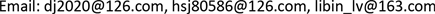JAJournal of Antennas2325-2227Scientific Research Publishing10.12677/JA.2018.73003JA-28280JA20180300000_24305027.pdf信息通讯 一种数字有源相控阵天线的测量方法 A New Method for Measuring Digital Active Phased Array Antenna 21书吉21立斌21中国电波传播研究所，电波环境特性及模化技术重点实验室，山东 青岛null2812201807031724© Copyright 2014 by authors and Scientific Research Publishing Inc. 2014This work is licensed under the Creative Commons Attribution International License (CC BY). http://creativecommons.org/licenses/by/4.0/Copyright © 2018 by authors and Hans Publishers Inc.1. 引言

2. 测量方法模型

E ( θ , ϕ ) = ∑ q = 1 N V q g u q ( θ , ϕ ) e j k r ^ r q (1)

E ( θ , ϕ ) = g a v i ( θ , ϕ ) ∑ n = 1 N i V n e j k r ^ r n + ∑ m = 1 N e V m g u m ( θ , ϕ ) e j k r ^ r m (3)

y = ∑ q = 1 N V q c q (2)

c q = e − j k d q d q g u q ( d ^ q ) ⋅ f p ( − d ^ q ) (3)

3. 变型的编码算法的测量原理

H 2 k = [ H 2 k − 1 H 2 k − 1 H 2 k − 1 − H 2 k − 1 ] (4)

a q = { t ( q ) V q ,         H ( m , n + 1 ) = + 1 V q ,                     H ( m , n + 1 ) = − 1 (5)

y ( m ) = ∑ q = 1 N a q c q = ∑ q = 1 N ( 1 + H ( m , n + 1 ) 2 V q + 1 − H ( m , n + 1 ) 2 t ( q ) w q ) c q (6)

H M − 1 = 1 M H M (7)

z ( q ) = 1 M ∑ m = 1 N H ( m , n + 1 ) y ( m ) ,       q = 1 , 2 , ⋯ , N (8)

z ( q ) = ( 1 − t ( q ) ) V q c q 2 ,       q = 1 , 2 , ⋯ , N (9)

V q = 2 ∗ z ( q ) ( 1 − t ( q ) ) c q ,       q = 1 , 2 , ⋯ , N (10)

Coding algorithms for three variant

V qV q exp ( j π )
V q exp ( j π )V q exp ( − j π / 2 )

V qα ∗ V q exp ( j π / 2 )
V q exp ( j π / 2 )α ∗ V q exp ( j π / 2 )

4. 仿真结果分析

5. 结论